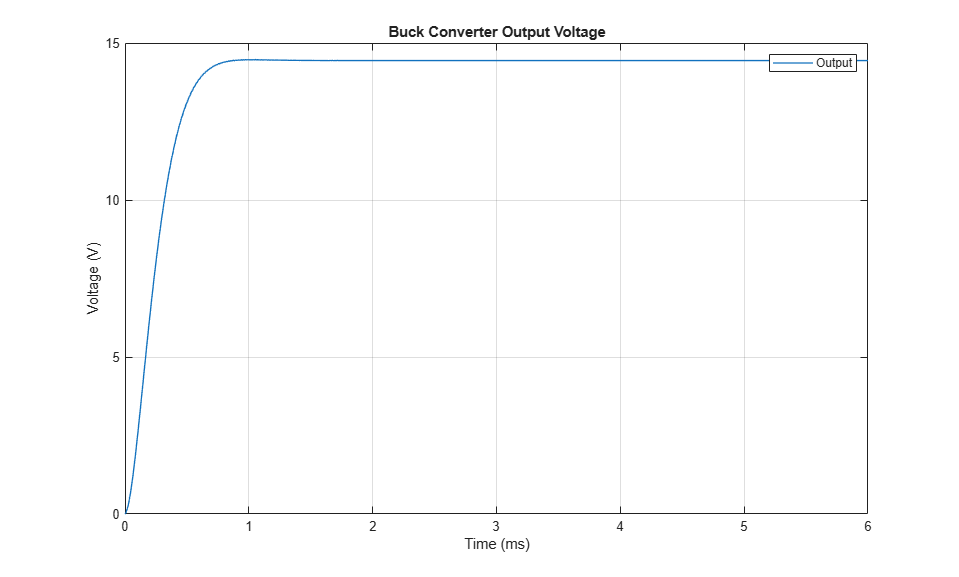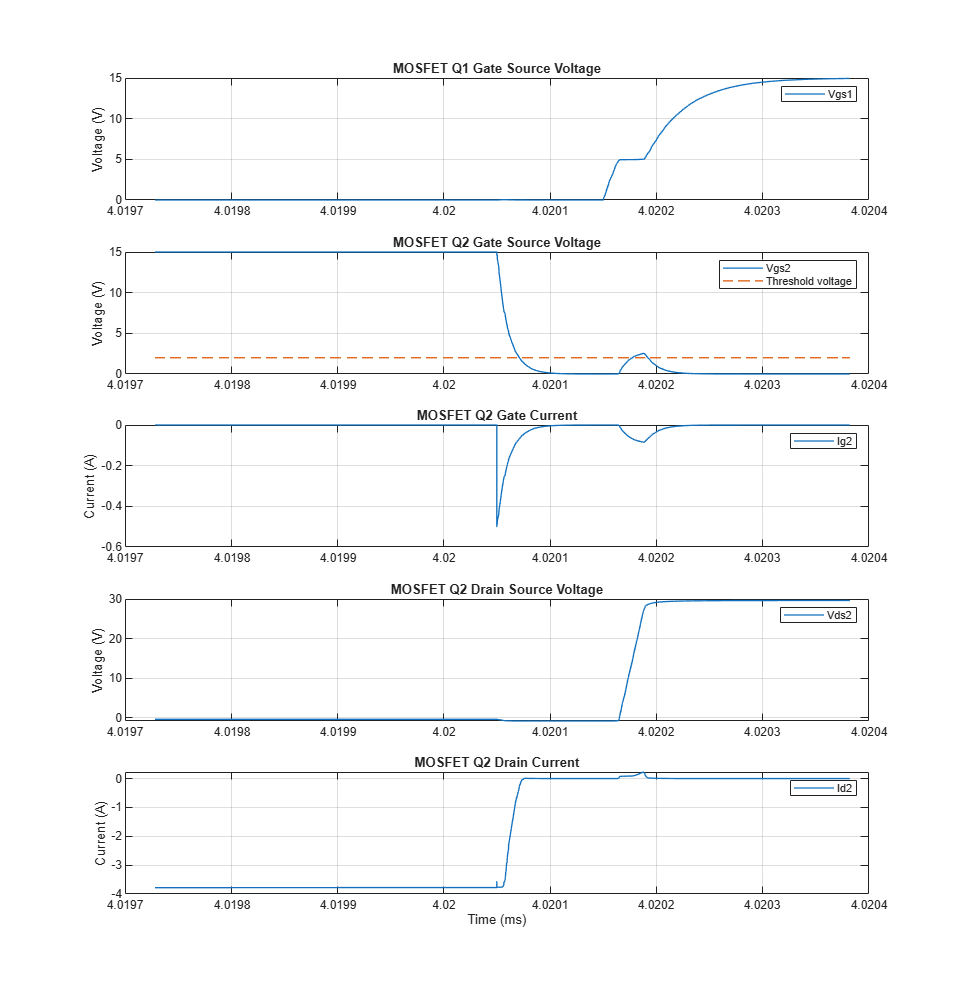# Analysis of Synchronous Buck Converter with Self Turn-On

This example analyzes the self turn-on mechanism in a synchronous buck converter. When the low-side MOSFET Q2 is off and the high-side MOSFET Q1 turns on, a current flows through the gate-drain capacitance of Q2 due to the voltage ramp dv/dt between the drain and source of Q2. This current induces a voltage across the gate and source of Q2. If the voltage exceeds the threshold voltage, Q2 unintentionally turns on. This example shows how the MOSFET parameters affect the self turn-on mechanism and how you can prevent it.

### Synchronous Buck Converter Overview### Simulation of Output Voltage and Self Turn-On

The plots below show the output voltage of the buck converter and illustrate the self turn-on mechanism of Q2. The duty cycle of the model is equal to 50%. In this example, to demonstrate the self turn-on phenomenon, the gate resistance is very high. Q2 self turns on at the rising edge of the gate source voltage of Q1.### Effect of Gate Resistance on High-Side MOSFET Q1

When Q1 turns on, its gate resistance affects the slope of the drain-source voltage of Q2. The higher gate resistance results in a lower slope rate of the drain-source voltage and reduces the occurrences of self turn-on for Q2.### Effect of Gate Resistance on Low-Side MOSFET Q2

The gate resistance of Q2 is directly proportional to its peak gate and to the time required to discharge the gate charge.### Effect of Gate Drain Capacitance of Low-Side MOSFET Q2

The current that flows through the gate-drain capacitance, Cgd, of Q2 is proportional to the gate-drain capacitance value. A small Cgd helps preventing the self turn-on mechanism of a low-side MOSFET.### Effect of Gate Source Capacitance of Low-Side MOSFET Q2

A high value of the gate-source capacitance, Cgs, decreases the peak gate source voltage of Q2 and increases the time required to discharge the gate charge. To prevent the self turn-on mechanism, as the gate-source voltage of Q2 is proportional to `Cgd/(Cgs+Cgd)`, use a MOSFET with low Cgd/Cgs ratio. However, high values of the gate-source capacitance reduce the switching speed.### Method of Preventing the Self Turn-On Mechanism

To prevent the MOSFET self turn-on mechanism, you should implement MOSFETs with high threshold voltage, low gate drain capacitance, and low gate drain to gate source capacitance ratio. You can add a capacitor between the gate and the source of the MOSFET to further reduce the Cgd/Cgs ratio.